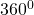# Cuboid

Cuboid: A solid whose, length, breadth & height are different is called a cuboid. It has a six rectangular plane faces, 12 edges & 8 vertex.
Cube: A cuboid whose length ,breadth & height are equal is called a cube.
Cylinder: When a rectangle. OABO’ is rotate at its oo’ axis in initial position tothus form a solid is called cylinder .
Cone: When a triangle AOV is rotate to its OV axis in initial position tothus form a solid is called cone.
Prism: When a traingle raise a required height H thus form a solid is called prism.
Cubes and cuboids are also known as square prism and rectangle prism.

Sphere: When a circle is rotate at its AOB axis in initial position tothus form a solid is called sphere.
Pyramid:A solid whose base is triangle or square in shape & it’s all vertices are meet a point at height H.
Triangular pyramid: A pyramid having triangular base is called triangular pyramid. He is also called a tetrahedeon (four faced sided )
Square pyramid: A pyramid having a square base is called a square pyramid.
Continued proportion e.g. 4:3::8:16
Mean proportion e.g. 4:2::8:16 or 82 = 4×16
8 is mean proportion.
Third proportion 4:8::8:16 – 16 is called third proportion.
Unitary method The method of finding is the value of one unit from the values of given own.